#### You may also like### Plants

Three children are going to buy some plants for their birthdays. They will plant them within circular paths. How could they do this?### Gran, How Old Are You?

When Charlie asked his grandmother how old she is, he didn't get a straightforward reply! Can you work out how old she is?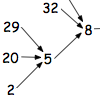What happens when you add the digits of a number then multiply the result by 2 and you keep doing this? You could try for different numbers and different rules.

# Polygonals

##### Age 7 to 11Challenge Level

Polygonal numbers are those that are arranged in shapes as they enlarge (starting with $1$). Here are examples of the $5$th polygonal numbers for the first $7$ shapes Triangle ($3$) through to Nonegon ($9$).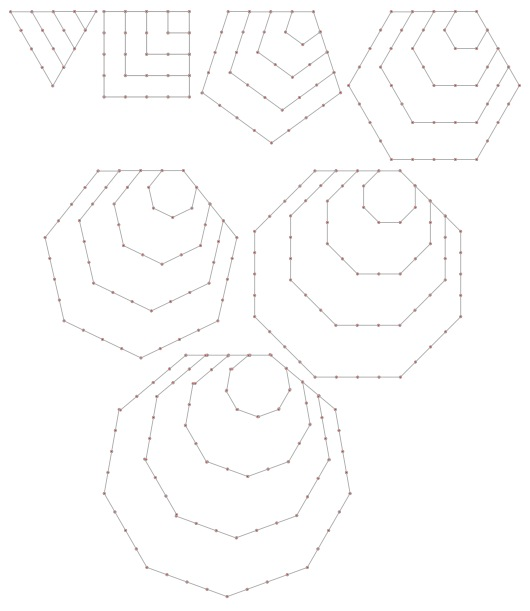If we were to just look at the hexagons we'd have a form of hexagonal numbers that grow  $1,6, 15, 28, 45$.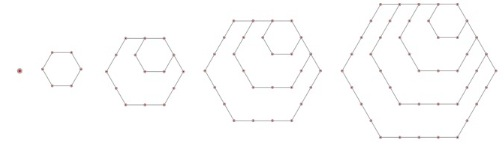There is another group that are called "Centred Polygonals"  that look like these;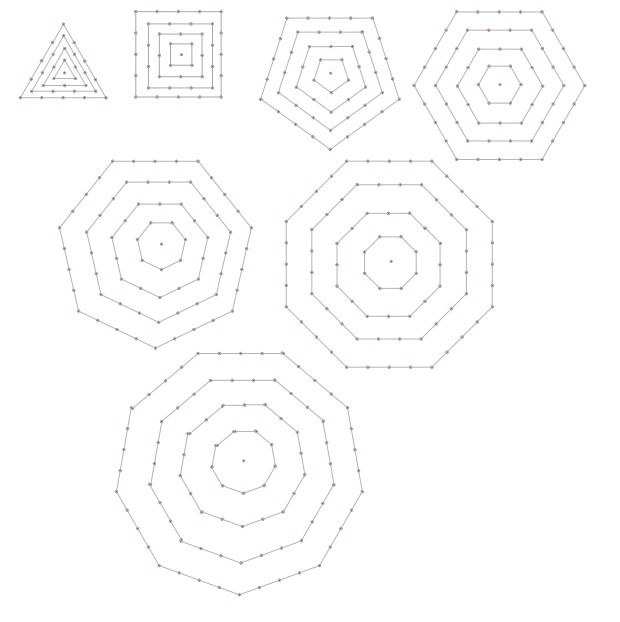and for example, the centred hexagon numbers go $1, 7, 19, 37, 61$.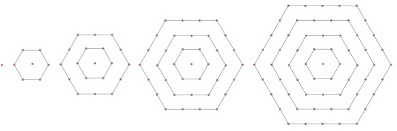#### Now it's over to you!

This investigation invites you to explore these sets of numbers and explore relationships within ordinary polygonal numbers and/or centred polygonal numbers.
You could also explore relationships between ordinary polygonal numbers and the centred polygonal numbers.

For example, you could explore which different polygonals (both centred and ordinary) have the same number occuring in the series?

KEY QUESTIONS:

What is the relationship between ordinary triangular polygonal numbers and others?

Can you re-arrange the dots from one polygonal to make another, and then generalise?